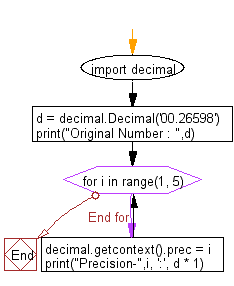﻿ Python Math: Round a specified decimal by setting precision - w3resource# Python Math: Round a specified decimal by setting precision

## Python Math: Exercise-40 with Solution

Write a Python program to round a specified decimal by setting precision (between 1 and 4).

Sample Solution:-

Python Code:

``````import decimal

d = decimal.Decimal('00.26598')
print("Original Number : ",d)
for i in range(1, 5):
decimal.getcontext().prec = i
print("Precision-",i, ':', d * 1)
```
```

Sample Output:

```Original Number :  0.26598
Precision- 1 : 0.3
Precision- 2 : 0.27
Precision- 3 : 0.266
Precision- 4 : 0.2660
```

Pictorial Presentation:Flowchart:Python Code Editor:

Have another way to solve this solution? Contribute your code (and comments) through Disqus.

What is the difficulty level of this exercise?

Test your Python skills with w3resource's quiz

﻿

## Python: Tips of the Day

Python: Get the Key Whose Value Is Maximal in a Dictionary

```>>> model_scores = {'model_a': 100, 'model_z': 198, 'model_t': 150}
>>> # workaround
>>> keys, values = list(model_scores.keys()), list(model_scores.values())
>>> keys[values.index(max(values))]
'model_z'
>>> # one-line
>>> max(model_scores, key=model_scores.get)
'model_z'
```